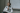# Exploring the Concept of Vertical Angles

POSTED ON APRIL 03, 2023Understanding the concept of vertical angles and the angles’ relationship gives you a chance to learn how to find unknown angle measures and relate pairs of angles. Get ready and learn how to spot vertical angles easily!

## What Are Vertical Angles?

Vertical angles are angles facing opposite each other at a point where two lines cross each other. When two lines intersect, four angles are formed. Each pair meets at the vertex. This is why each pair of angles are called vertical angles, from the word, vertex.Take a look at the pair of angles, and , formed by two intersecting lines. These two meet at the vertex, O, and are facing opposite each other. Hence, the two angles are a pair of vertical angles.

Juni Learning has excellent resources on fundamental geometry such as intersecting lines and vertical angles. They offer a safe space for you to explore new techniques and methods in a holistic approach. Explore Juni Learning’s content to support you in mastering Common Core math concepts – plus other subjects too!

Online Tutoring with Juni Tutor Bot - Get Free Help 24/7
Juni Tutor Bot is your friendly online tutoring assistant. Get immediate, free homework help and exam support for general math, calculus, algebra tutoring, and standardized test prep like SATs. Learn with a community of peers and tutors, supported by AI.

When it comes to vertical angles, always remember: vertical angles are equal. This means that the pair of angles, and , have equal angle measures.Can you identify two pairs of angles just by looking at the figure above? Focus on the angles that face opposite each other:

• First pair of vertical angles: ∠AOC and ∠BOD face opposite each other.
• Second pair of vertical angles: ∠AOB and ∠COD meet at the vertex O.

As you can see from the figure, each pair of angles will have equal angle measures.Don’t forget this golden rule for vertical angles: their measures are equal. Use this to answer the sample problem below!

#### Example 1

Using the figure shown below, what is the value of x?The two angles, 64° and , face opposite each other between two intersecting lines. Hence, these two are vertical angles. Applying the golden rule for vertical angles, their angle measures must be equal.

64° = x°

x = 64

Hence, x is equal to 64.